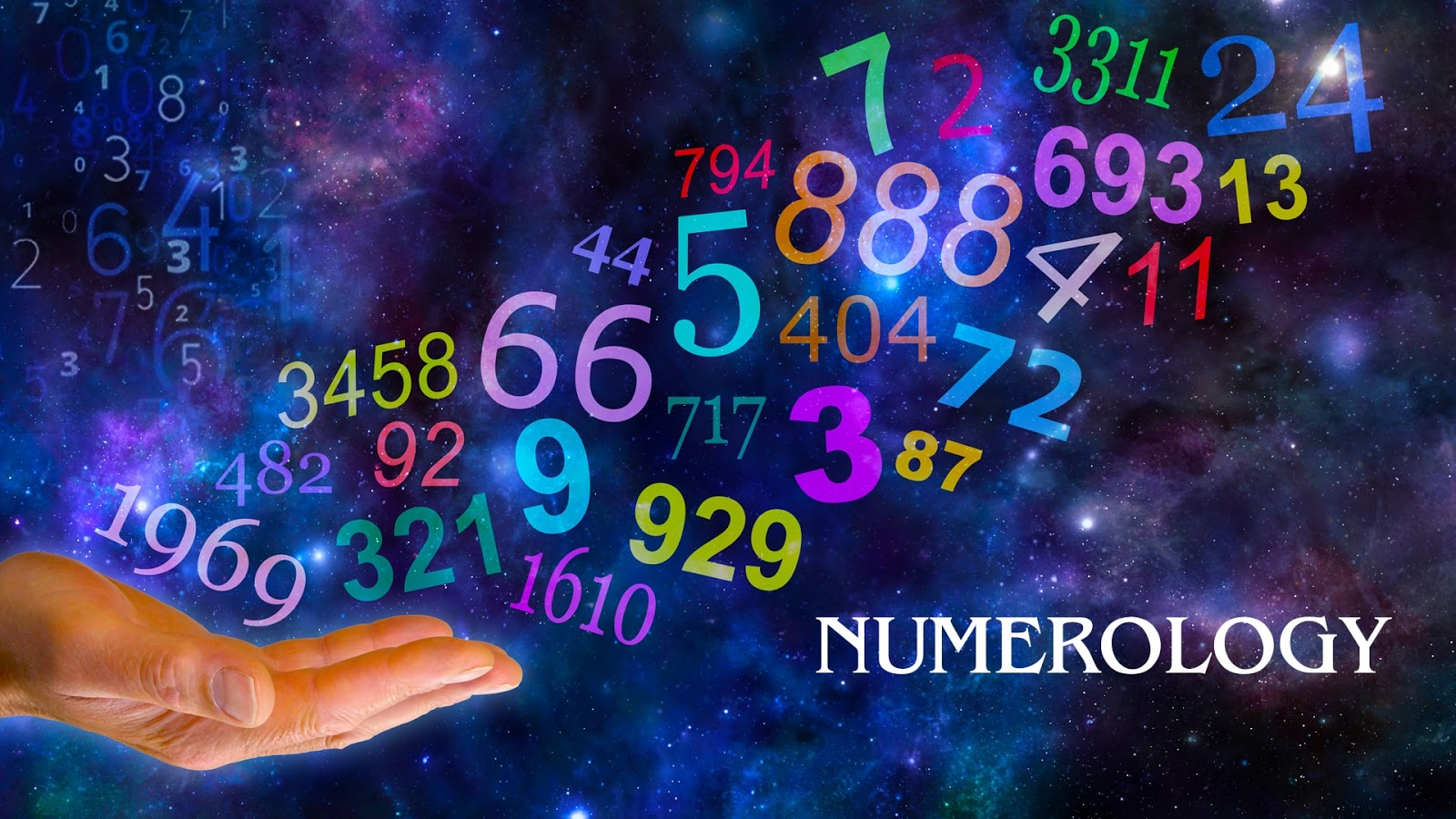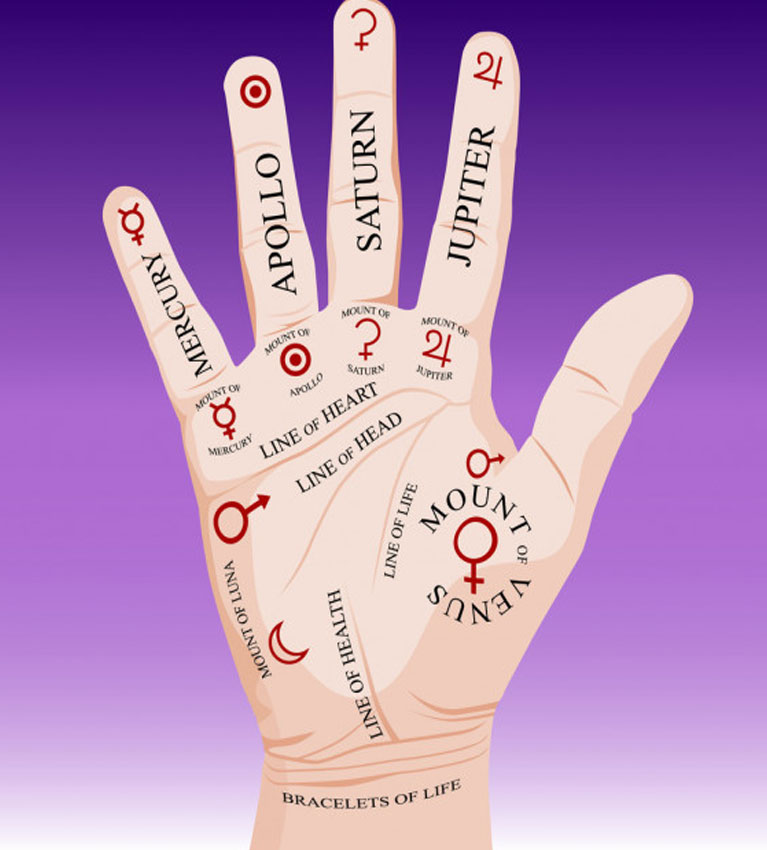# Numerology

April 29, 2014###### Palmistry
April 28, 2014April 30, 2014

#### WHAT IS NUMEROLOGY?

According to the science of numerology, it is a belief that there’s a mystical link between the events happening around you and the numbers that correspond to your name or date of birth. As stated by the Astro Jagannath , this particular stream of science opines that numbers together, form a universal language, which actually, in turn, confirms the truth of a person’s life. These numbers determine the momentum of your ‘Life Path” and are valid as the natural elements – air, water, fire, and earth surrounding you, or even the topographies and climatic conditions. Each and every individual has a unique Life Path number, which is also the most important aspect of his natal chart. Life Path Number is that very number and the key to the person’s inner self, his characteristic traits, habits, behavior, and relationship with other individuals. In numerology, the numbers that speak for an individual run from 1 through 9 but there are also exceptions. For instance, the figures 11, 22, 33, 44, 55, 66, 77, 88, 99 are called ‘Master Numbers,’ which should not be added to obtain a single digit.### „HERE ARE SOME OF THE TIPS GIVEN BY THE GREAT ASTROLOGER IN INDIA TO CALCULATE LIFE’S PATH BASED ON YOUR NAME:”

#### For instance, “A” to “I” will bear the numbers 1 to 9. This will be repeated for “J” to “R”. Lastly, “S” to “Z First of all, you have to assign the numbers 1-9 repeatedly for each of the numbers in the alphabet.” will bear the numbers 1 to 8. Now, you will have the following groups:

• 1 = A, J, S.
• 2 = B, K, T.
• 3 = C, L, U.
• 4 = D, M, V.
• 5 = E, N, W.
• 6 = F, O, X.
• 7 = G, P, Y.
• 8 = H, Q, Z.
• 9 = I, R.
Now, if your name is Narendra Modi, your Life Path numbers can be calculated as follows: N(5) + A(1) + R(9) + E(5) + N(5) + D(4) + R(9) + A(1) + M(4) + O(6) + D(4) + I(9) = 62 = 6 + 2 = 8 You have to use your full name for the purpose. Using only your first name, middle name, surname, nickname, or a name post affidavit can lead to different results and different interpretations.

##### Few tips by the best numerologist in India in order to calculate life path number based on birth date :

Birth date is the most important number of all; it complies with the natal chart as well. In order to calculate the Life Path Number of an individual based on his date of birth, follow the given steps:
• Add the digits in each unit (viz. month, date, and year) until you have a single-digit or Master Number for each. For instance, if a person’s date of birth is 11th August, 1986, you should take into account the following: Month – August = 8; Date – 11, i.e. 1+1 = 2; Year – 1987 means 1+9+8+6 = 24; 2+4 = 6.
• Now add up the results, i.e. 8+2+6 = 16. 1+6 = 7.
• Thus, the Life Path Number is 7.
• Numbers like 11, 22, 33, 44, 55, 66, 77, 88, and 99 should not add to get a single digit as those are ‘Master Numbers’.#### Numerology and the Nine Planets:

Numerology also shares a deep bond with the nine planets in our solar system. Each planet responds to the great Zodiac and the twelve signs defined by it. Thus, each planet determines specific traits of an individual. Let’s check out which is what.

• The Sun: Positive for 1.
• The Moon: Positive for 2.
• Mars: Positive for 9.
• Venus: Positive for 6.
• Mercury: Positive for 5.
• Jupiter: Positive for 3.
• Saturn: Positive for 8.
• Rahu: Positive for 4.
• Ketu: Positive for 7.

#### Numerology and the Days of The Week

Each and every individual with a unique number is also assigned to each of the days in a week. The assignment, however, is overlapping. The numbers according to the weekdays are –
• Sunday: 1-4.
• Monday: 2-7.
• Tuesday: 9.
• Wednesday: 5.
• Thursday: 3.
• Friday: 6.
• Saturday: 8.

##### Compatibility Based On Numbers:

• 1: 1, 5, 7.
• 2: 2, 4, 8.
• 3: 3, 6, 9.
• 4: 2, 4, 8.
• 5: 1, 5, 7.
• 6: 3, 6, 9.
• 7: 1, 5, 7.
• 8: 2, 4, 8.
• 9: 3, 6, 9.